### Linear Vector Spaces: Basic Definitions

#### Learning Outcomes

• Identify the dimension of a linear vector space
• Identify the basis of a linear vector space
• Identify whether vectors are linearly dependent or not

### Linear Vector Space

A set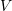is called a linear vector space over the field of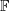if the two operations (vector addition)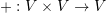and (scalar multiplication)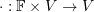are defined and satisfy the following 8 axioms: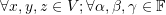:

1. Commutativity: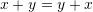2. Associativity: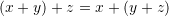3. Distributivity of vector addition over scalar multiplication: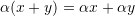4. Distributivity of scalar addition over scalar multiplication: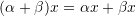5. Compatibility of scalar multiplication with field multiplication: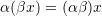6. Identity element of addition/Zero element: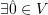such that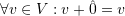7. Inverse element of addition: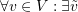such that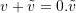is denoted8. Identity element of scalar multiplication: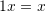The elements of the linear vector space are called vectors, while the elements ofare called scalars. In general,is either the field of real numbers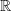or of complex numbers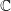. For the remainder of this article,is assumed to be the field of real numbersunless otherwise specified.

In the history of the development of linear vector spaces, the following statements sometimes appeared in the definition. However, the above 8 essential axioms can be used to prove them.

• The element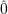is unique.
•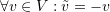is unique.
• If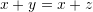, then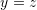.
•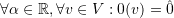and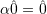.
•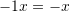Notice that in the above definition and statements, the hat symbol was used to distinguish the zero vectorfrom the zero scalar 0. However, in the future, 0 will be used for both and it is to be understood from the context whether it is the zero vector or the zero scalar.

Examples of linear vector spaces include the following sets:

••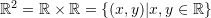•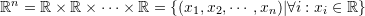• The set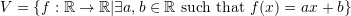is a linear vector space!

### Subspaces

A subset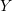of a vector spaceis called a subspace if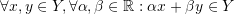.
In other words, a subsetof a vector spaceis called a subspace if it is closed under both vector addition and scalar multiplication. For example, the set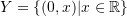is a subspace of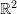(why?).

### Linear Independence

Let V be a linear vector space over. A set of vectors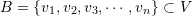is called a linearly independent set of vectors if none of the vectors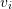can be expressed linearly in terms of the other elements in the set; i.e., the only linear combination that would produce the zero vector is the trivial combination: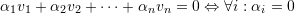Otherwise, the set is called linearly dependent.

Examples:
Consider the vector space. The set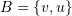where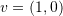and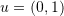is linearly independent: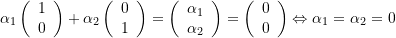However, the set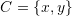where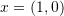and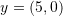is linearly dependent: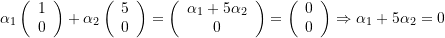This means that there are multiple combinations that would give the zero vector. For example, choosing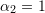and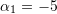would yield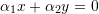.

Notice that if the zero vector is an element of a set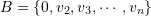then, the set is automatically linearly dependent since the following non trivial combination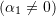would produce the zero vector: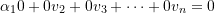### Basis and Dimensions

A subset B of a linear vector space V is called a basis set if it is a set of linearly independent vectors such that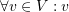can be expressed as a linear combination of the elements of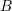. Alternatively: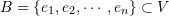is a basis ofif and only if the following two conditions are satisfied:

•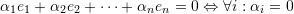•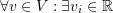such that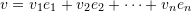The dimension of a linear vector space V is the number of elements in its basis set.
For example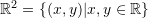is a two dimensional space, because its basis set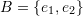has two elements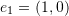and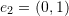.

Assertion: Ifis a basis set of, then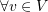the combinationis unique. i.e.,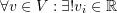such that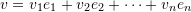.

Proof: Use the linear independence of the basis setto prove the result.

Click on the image below to interact with this example. Input the components of the vectors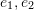and. The tool draws the vectors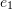and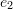in blue and red respectively. It identifies if the vectors are linearly dependent or linearly independent. If they are linearly independent, the tool calculates and draws the components ofin the directions ofand.